modEvA is an R package for analysing and evaluating species distribution models. Most functions are meant for generalized linear models (GLMs) with binomial distribution and a logit link function (i.e., logistic regression), although many can be applied to other models as well. Most functions can also be applied to values of observed and corresponding predicted values (both in a table or as raster predictions and presence point observations), as long as they are bounded between 0 and 1.

The modEvA package works within the free and open-source R statistical software, so you first need to download, install and open R (available at http://www.r-project.org). In this tutorial, in `monospaced` font are the commands that you need to type (or copy and paste) into the R console (and then press the enter key to execute them). For commands that generate visible results in R, these are usually shown below them, preceded by hash marks (##). Note that all commands are case-sensitive, so you must respect upper- and lower-case letters; that you must always use straight (`'`, `"`) rather than curly quotes and apostrophes; and that R is only ready to receive a new command when there’s a prompt sign (>) at the end of the R console; if not, it’s still waiting for an operation to be finished or for you to complete a previous command – watch out for unclosed parentheses and such.

Install the latest version of modEvA by pasting the command below in the R console (when connected to the internet):

``install.packages("modEvA", repos="http://R-Forge.R-project.org")``

You only need to install the package once (unless a new version becomes available), but you need to load it every time you open a new R session in which you intend to use modEvA (no need for an internet connection anymore), by pasting the following command in R:

``library(modEvA)``

## Analysing models

Load the rotif.mods sample dataset (based on a global dataset of rotifer distribution records originally published with this article) to use as an example:

``data(rotif.mods)``

You can get more information on this dataset (the following command should open an R Documentation window):

``help(rotif.mods)``

You can see that rotif.mods is a list containing two elements: a list of models and a dataframe with their predictions. Let’s leave the predictions alone for now, and work on the model objects. Let’s start by checking out their names:

``names(rotif.mods\$models)``
``````##   "Abrigh" "Afissa" "Apriod" "Bangul" "Bcalyc" "Bplica" "Bquadr" "Burceo"
##   "Cgibba" "Edilat" "Flongi" "Kcochl" "Kquadr" "Ktropi" "Lbulla" "Lclost"
##  "Lhamat" "Lluna"  "Llunar" "Lovali" "Lpatel" "Lquadr" "Mventr" "Ppatul"
##  "Pquadr" "Pvulga" "Specti" "Tpatin" "Tsimil" "Ttetra"``````

These names correspond to species codes, and each object in this list is a GLM of the presence-absence of the corresponding species. Now let’s assign the first model to an individual object, and use it to try out modEvA functions. The following alternate commands should both create a model object named “mod” from the 1st model in the rotif.mods\$models list, which is named “Abrigh”:

``````mod <- rotif.mods\$models[]
mod <- rotif.mods\$models[["Abrigh"]]``````

In most modEvA functions, instead of a model object, you can provide vectors of observed and predicted values, or presence points and raster predictions, that you’ve obtained elsewhere. Let’s extract such values from this model, to be able to use them in the examples as well:

``mydata <- data.frame(observed = mod\$y, predicted = mod\$fitted.values)``

Let’s now try modEvA functions on this model’s predictions (or you can use any other GLM object or observed-predicted values that you may have). Let’s start with function plotGLM, which shows how observed (grey) and predicted (black) values vary along the regression equation:

``plotGLM(model = mod, xlab = "Logit (Y)", ylab = "Predicted probability", main = "Model plot")``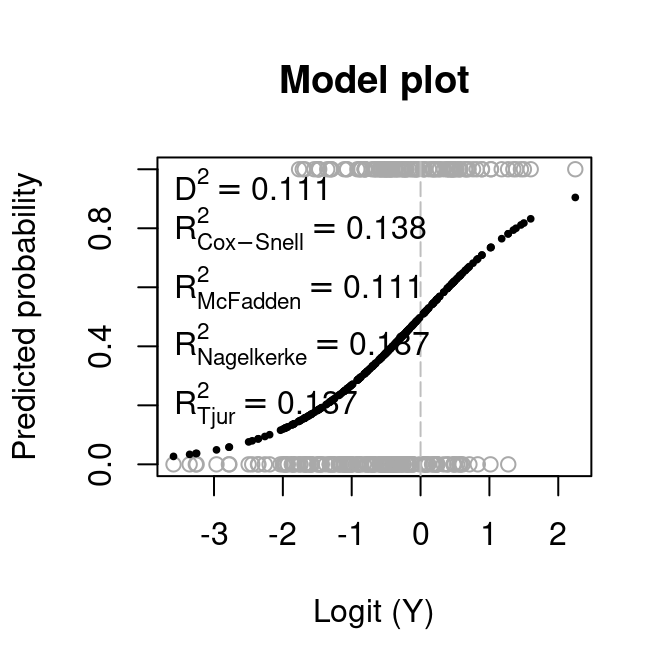Instead of the model object, you can provide your observed and predicted values:

``plotGLM(obs = mydata[ , "observed"], pred = mydata[ , "predicted"], xlab = "Logit (Y)", ylab = "Predicted probability", main = "Model plot")``

## Evaluating discrimination capacity

Let’s now calculate the area under the ROC curve (AUC) for this model. The AUC function produces the plot displayed below and also some text results, which will appear in your R console but will not be shown here. Again, as in most modEvA functions, you can provide obs = mydata[ , “observed”], pred = mydata[ , “predicted”] instead of model = mod; or you can provide a raster map of your predictions and a set of spatial points of the presence observations to compare these predictions with.

``AUC(model = mod, main = "ROC curve")``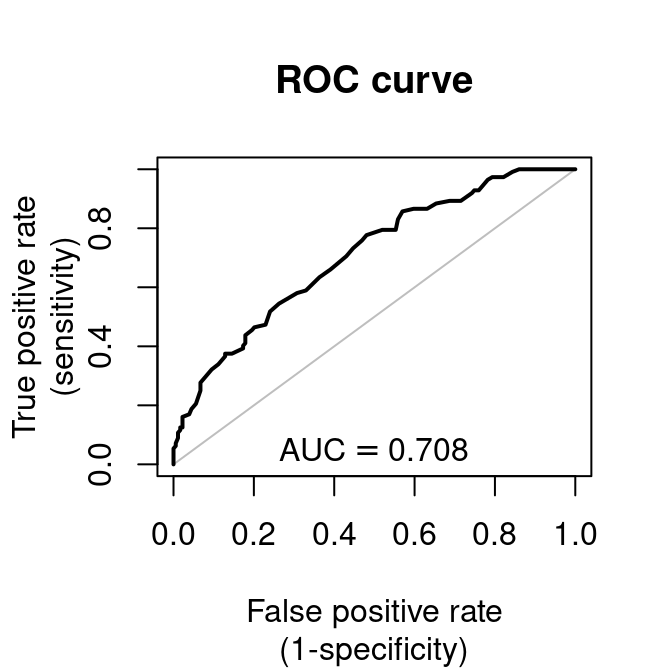You can also plot and compute the area under the precision-recall curve:

``AUC(model = mod, curve = "PR", main = "Precision-Recall curve")``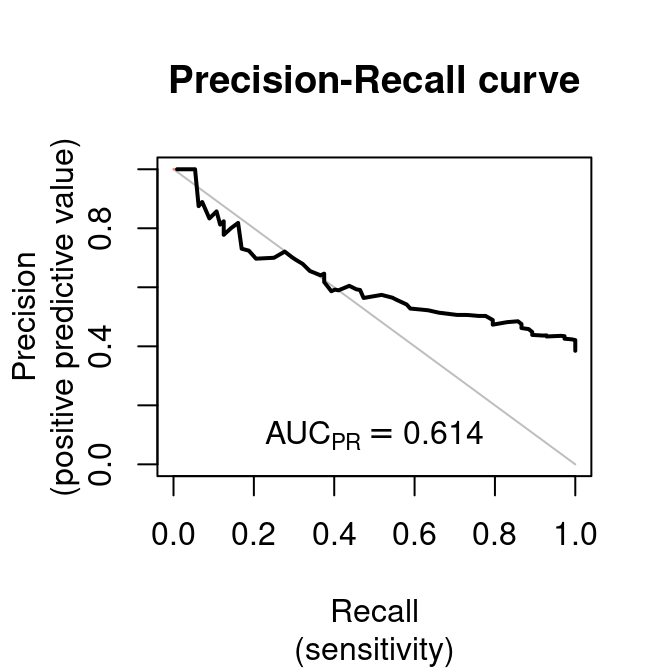Now compute some threshold-based evaluation measures for this model, using maximum TSS (i.e. the best balance between sensitivity and spcificity) as the threshold value above which to consider that the model predicts the species to be present. Only the plot is shown here, but additional text results should appear in your R console as well:

``````par(mar = c(5.6, 4.1, 2, 2.1))  # changes figure margins
threshMeasures(model = mod, thresh = "maxTSS", ylim = c(0, 1), main = "Threshold measures")``````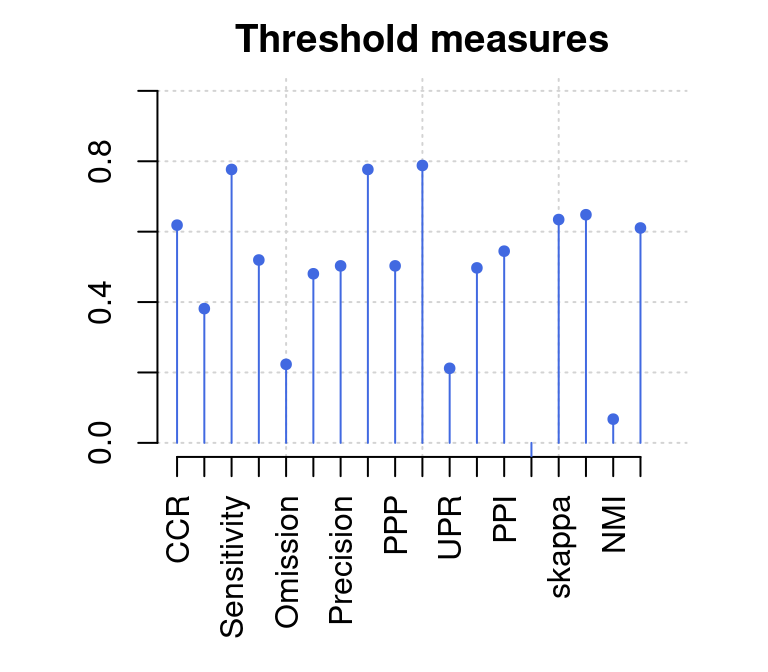Note that there are many different criteria for choosing the `thres`hold value for a model (see Details in `help(threshMeasures)`). Let’s see how each threshold-based measure varies along with the chosen prediction threshold, to identify optimal thresholds according to different criteria:

``optiThresh(model = mod, pch = 20, cex = 0.2)``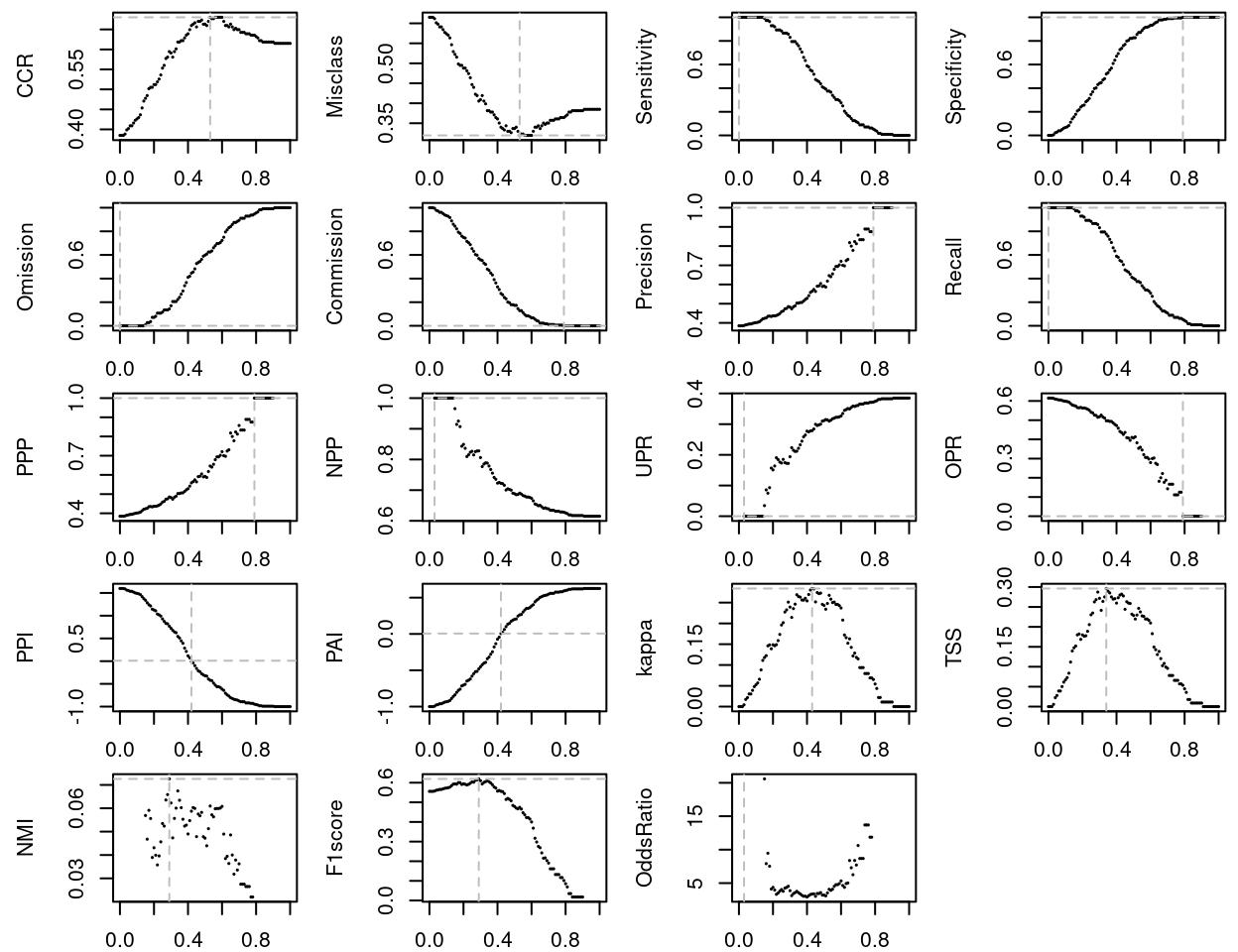You can also compute the optimal threshold balancing two complementary evaluation measures:

``optiPair(model = mod, measures = c("Sensitivity", "Specificity"), main = "Optimal balance")``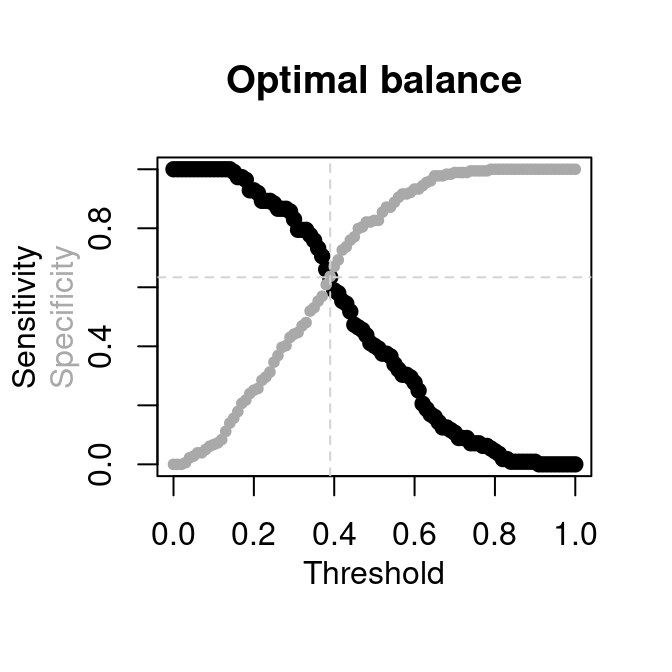Not that the above is equivalent to maximizing TSS, which also balances sensitivity and specificity. You can try the same command with other pairs of related measures, such as `c("Omission", "Commission")`, `c("PPI", "PAI")`, etc..

## Assessing model explanatory power, fit and calibration

Let’s now assess the proportion of variation that the model accounts for. For GLMs there isn’t a single consensual measure for this; modEvA can calculate the explained deviance (D-squared), optionally adjusted for the number of observations and parameters; and some pseudo R-squared values (see `help(Dsquared)`and `help(RsqGLM)` for further info):

``Dsquared(model = mod)``
``##  0.1114199``
``Dsquared(model = mod, adjust = TRUE)``
``##  0.09264705``
``RsqGLM(model = mod, ylim = c(0, 0.4), main = "R-squared values")``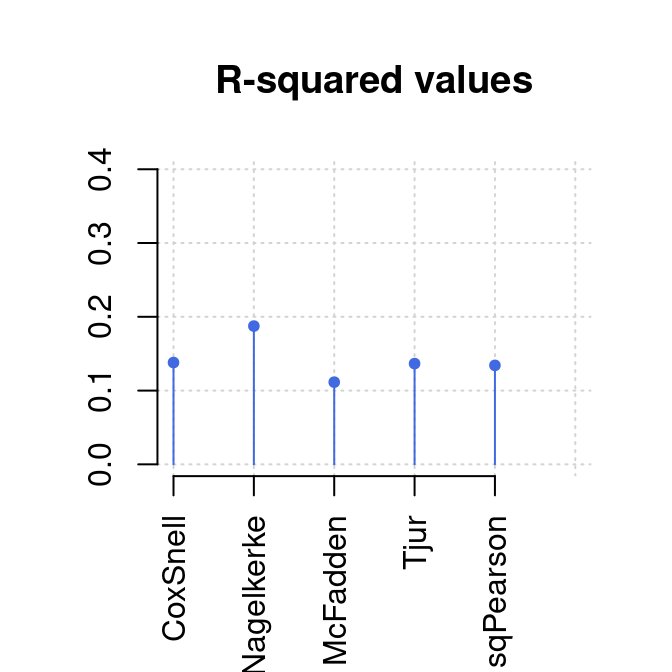``````## \$CoxSnell
##  0.1380008
##
## \$Nagelkerke
##  0.187434
##
##  0.1114199
##
## \$Tjur
##  0.1365661
##
## \$sqPearson
##  0.134168``````

We can also take a look at some model calibration measures, such as Miller’s calibration statistics, the Continuous Boyce Index and the Hosmer-Lemeshow goodness-of-fit. Note, however, that the first one is not useful for evaluating a model on the same data used for building it (the results will look perfect by definition); and that the latter two can depend strongly on the bin.method used for grouping the values. See `help(MillerCalib)`, `help(Boyce)` and `help(HLfit)` to find out how these measures are calculated and how their results can be interpreted.

``MillerCalib(model = mod)``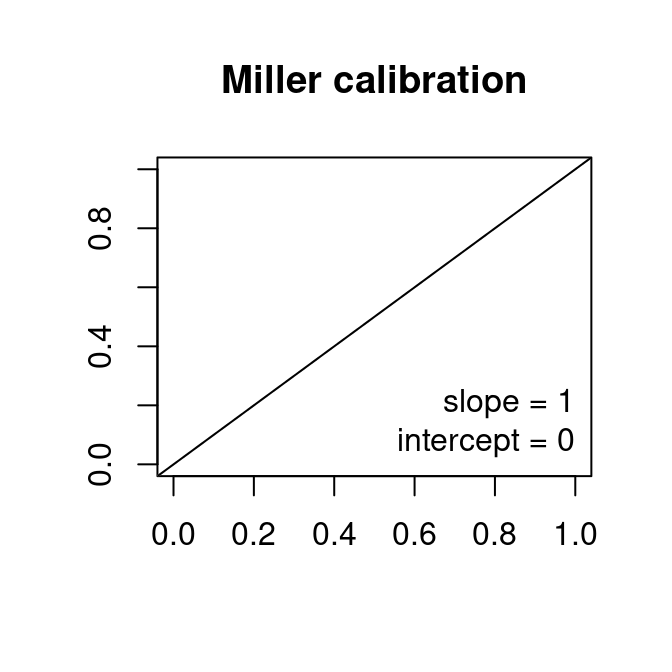``Boyce(model = mod, bin.method = "quantiles", main = "Boyce index, default")``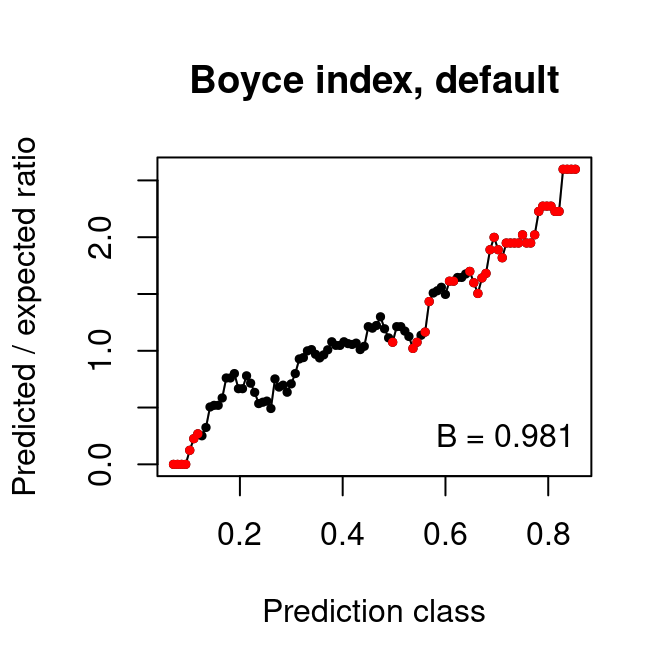``Boyce(model = mod, bin.width = 0.2, main = "Boyce index, 0.2-size bins")``